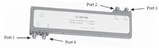# 180 Degree Hybrids Testing And Term Definitions

Source: KrytarWhen a signal is applied to port 1, the output signal will appear on ports 2 and 3. Ports 2 and 3 are 180 degrees out of phase with each other.

When a signal is applied to port 1, the output signal will appear on ports 2 and 3. Ports 2 and 3 are 180 degrees out of phase with each other.

When a signal is applied to port 4, the output signal will appear on ports 2 and port 3. Ports 2 and 3 are in phase with each other.

AMPLITUDE IMBALANCE MEASUREMENT
The specification for Amplitude Imbalance for Model 4010180 is ±0.6 dB. Krytar measures amplitude imbalance from output port 3 to port 2 (0.0 dB reference) with a vector analyzer. Note maximum deviation from the zero reference is approximately -0.6 db which appears at approximately 7.5 GHz. Because there is no corresponding positive peak at the specific frequency of 7.5 GHz, the allowable total deviation at that specific frequency would then be 1.2 dB. The definition for amplitude imbalance of Krytar Model 4010180 3 dB 180 degree hybrid is: 1.2 dB maximum from the 0.0 dB reference at any specific frequency point within the frequency band of 1.0 to 18.0 GHz.:

INSERTION LOSS MEASUREMENT
The specification for Insertion Loss for Model 4010180 is 2.9 dB maximum. The insertion loss is measured from Port 1 to Port 2 and for Port 1 to Port 3 using a vector analyzer. Insertion loss from Port 2 is superimposed on Insertion loss of Port 3. Note that maximum insertion loss over the entire frequency band is approximately 1.83 dB.